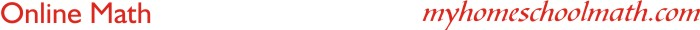Order of operations

 Example 1: 35 + 8 x 5 = 35 + (8 x 5) = 35 + 40 = 75 Example 2: 20 x 4 - 2 = (20 x 4) - 2 = 80 - 2 = 78